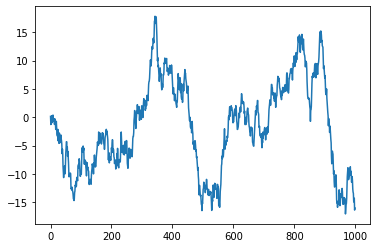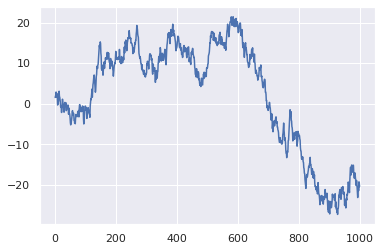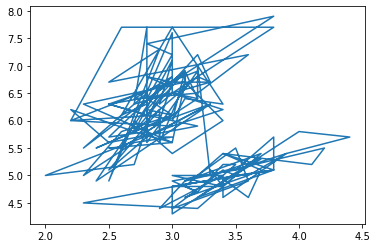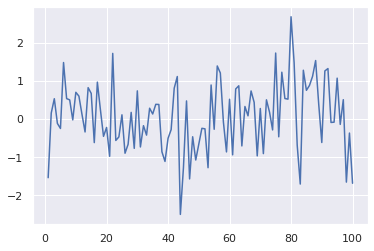# Line chart with matplotlib

A line chart or line graph is a type of chart which displays information as a series of data points called ‘markers’ connected by straight line segments. It is similar to a scatter plot except that the measurement points are ordered (typically by their x-axis value) and joined with straight line segments. This post will show how to plot a basic line chart using matplotlib.

## Basic Lineplot

You can create a basic line chart with the `plot()` function of matplotlib library. If you give only a serie of values, matplotlib will consider that these values are ordered and will use values from 1 to n to create the X axis (figure 1):

``````# libraries
import matplotlib.pyplot as plt
import numpy as np

# create data
values=np.cumsum(np.random.randn(1000,1))

# use the plot function
plt.plot(values)

# show the graph
plt.show()``````## Seaborn Customization

For a more trendy look, you can use the `set_theme()` function of seaborn library. You will automatically get the look of figure 2.

``````# libraries
import matplotlib.pyplot as plt
import numpy as np
import seaborn as sns

# use seaborn style
sns.set_theme()

# create data
values=np.cumsum(np.random.randn(1000,1))

# use the plot function
plt.plot(values)

# show the graph
plt.show()``````## Use lineplot with unordered data

You can also make a line chart from 2 series of values (X and Y axis). However, make sure that your X axis values are ordered! If not, you will get this kind of figure (figure 3).

``````# libraries
import matplotlib.pyplot as plt
import seaborn as sns

# import the iris dataset

# plot
plt.plot( 'sepal_width', 'sepal_length', data=df)

# show the graph
plt.show()``````## Use lineplot with ordered data

If your X data is ordered, then you will get a similar figure as figure 1:

``````# libraries and data
import matplotlib.pyplot as plt
import numpy as np
import seaborn as sns
import pandas as pd
df=pd.DataFrame({'xvalues': range(1,101), 'yvalues': np.random.randn(100) })

# plot
plt.plot( 'xvalues', 'yvalues', data=df)

# show the graph
plt.show()``````## Contact & Edit

👋 This document is a work by Yan Holtz. Any feedback is highly encouraged. You can fill an issue on Github, drop me a message onTwitter, or send an email pasting `yan.holtz.data` with `gmail.com`.